Courses

# Revisal Problems (Past 13 Years) JEE Advanced (Thermodynamics)

## 20 Questions MCQ Test Chemistry for JEE Advanced | Revisal Problems (Past 13 Years) JEE Advanced (Thermodynamics)

Description
This mock test of Revisal Problems (Past 13 Years) JEE Advanced (Thermodynamics) for JEE helps you for every JEE entrance exam. This contains 20 Multiple Choice Questions for JEE Revisal Problems (Past 13 Years) JEE Advanced (Thermodynamics) (mcq) to study with solutions a complete question bank. The solved questions answers in this Revisal Problems (Past 13 Years) JEE Advanced (Thermodynamics) quiz give you a good mix of easy questions and tough questions. JEE students definitely take this Revisal Problems (Past 13 Years) JEE Advanced (Thermodynamics) exercise for a better result in the exam. You can find other Revisal Problems (Past 13 Years) JEE Advanced (Thermodynamics) extra questions, long questions & short questions for JEE on EduRev as well by searching above.
QUESTION: 1

Solution:
QUESTION: 2

Solution:
QUESTION: 3

### A 50.0 kg person drinks 500 g of milk which has a calorific value of approximately 720 cal g-1. If only 10% of the energy in milk is converted to mechanical work, how high (in meters) can the person climb based on this energy intake?

Solution:
QUESTION: 4

For the reaction,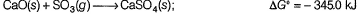Q. ΔG for this reaction at 298 K when the pressure of SO3 is 0.5 atm is

Solution:
QUESTION: 5

If the internal energy of a system decreases by 125 J and at the same time it absorbs 54 J of heat then

Solution:
QUESTION: 6

In compressing a gas, 355 J of work is done on the system. At the same time 185 J of heat escapes from the system. Thus, ΔE for the system is

Solution:
QUESTION: 7

0.4 mole of xenon is heated from 300 K to 400 K. Thus, change in internal energy is approximately (assume ideal behaviour and heat capacity is independent of temperature)

Solution:
QUESTION: 8

Direction (Q. No. 8) This section is based on Statement I and Statement II. Select the correct answer from the codes given below.

Q.

Statement I : When liquid water freezes under constant pressure, work is done by the system.

Statement II : When liquid water freezes, volume increases.

Solution:
*Multiple options can be correct
QUESTION: 9

Direction (Q. Nos. 9-11) This section contains 3 multiple choice questions. Each question has four choices (a), (b), (c) and (d), out of which ONE or MORE THAN ONE are correct.

Q. The standard enthalpies of combustion of fumaric acid and maleic acid are -1336.0 and -1359.2 kJ mol-1

ΔfH° (CO2) = - 393.5 kJ mol-1
ΔfH° (H2O) = - 285.8 kJ mol-1

Solution:
*Multiple options can be correct
QUESTION: 10

Select correct alternates based on the following experiment.

A quantity of 0.850 mole of an ideal gas initially at a pressure 15.0 atm and 300 K is allowed to expand isothermally until its final pressure is 1.00 atm. Work done

Solution:
*Multiple options can be correct
QUESTION: 11

A quantity of 200 mL of 0.862 M HCI is mixed with 200 mL of 0.431 M Ba(OH)2 in a constant pressure calorimeter that has a heat capacity of 453 JK-1. The initial temperature of the HCI and Ba(OH)2 solution is the same at 20.48°C. For the process the heat of neutralisation for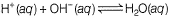is -56.2 kJ mol-1.

Select correct statement(s)

Solution:
QUESTION: 12

Direction (Q. Nos. 12-17) This section contains 3 paragraphs each describing theory, experiments, data etc. Six questions related to the paragraphs have been given. Each question has only one correct answer among the four given options (a), (b), (c) and (d).

Passage I

The combustion of methane gas, the principal constituent of natural gas is represented by the equation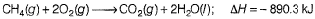Q. Quantity of heat, in kilojoules, liberated in the complete combustion of 1.65 x 104 L of CH4(g) measured at 27°C and 760 mm Hg is

Solution:
QUESTION: 13

Passage I

The combustion of methane gas, the principal constituent of natural gas is represented by the equationQ. Volume of water, in litres, that could be heated from 8.8°C to 68.8°C as a result of above heat is (density = 1 g/mL

Solution:
QUESTION: 14

Passage II

A system consisting of 1 mole of water vapour H2O(g) at 100°C and 1 atm with a volume of 30.12 L and condenses at a constant pressure of 1 atm to liquid water, H2O(l) at 100°C and 1 atm with a volume of 18.8 cm3.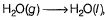ΔH = -40.7 kJ

Q. Work done in this transformation is

Solution:
QUESTION: 15

Passage II

A system consisting of 1 mole of water vapour H2O(g) at 100°C and 1 atm with a volume of 30.12 L and condenses at a constant pressure of 1 atm to liquid water, H2O(l) at 100°C and 1 atm with a volume of 18.8 cm3.ΔH = -40.7 kJ

Q. ΔE of the process is

Solution:
QUESTION: 16

Passage III

Based on the following figure.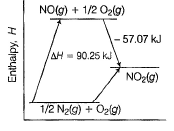Q. ΔfH of NO2(g) is

Solution:
QUESTION: 17

Passage III

Based on the following figure.Q. Heat of combustion of NO(g) is

Solution:
QUESTION: 18

Direction (Q. No. 18) Choices for the correct combination of elements from Column I and Column II are given as options (a), (b), (c) and (d), out of which one is correct.

Q. Acetic acid, CH3CO2H can form dimer (CH3CO2H)2 in the gas phase.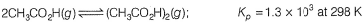The dimer is held together by two hydrogen bonds with a total strength of 66.5 kJ mol-1 of dimer.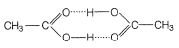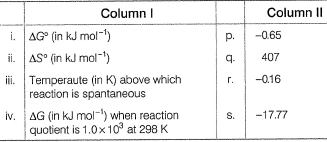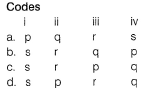Solution:
*Answer can only contain numeric values
QUESTION: 19

Direction (Q. Nos. 19 and 20) This section contains 2 questions. Each question, when worked out will result in integer from Oto 9 (both inclusive).

Q. A quantity of 0.50 mole of an ideal gas at 300 K expands isothermally against a constant pressure of 2.0 atm from 1.0 L to 5.0 L. Thus ΔS (universe) is .......

Solution:
*Answer can only contain numeric values
QUESTION: 20

The molar heat capacity of oxygen at constant pressure is given by (25.7T + 0.01307) 10-3 kJ mol-1.

Calculate the enthalpy change (in kJ) when 1.45 moles of O2 are heated from 298 K to 367 K.

Solution: